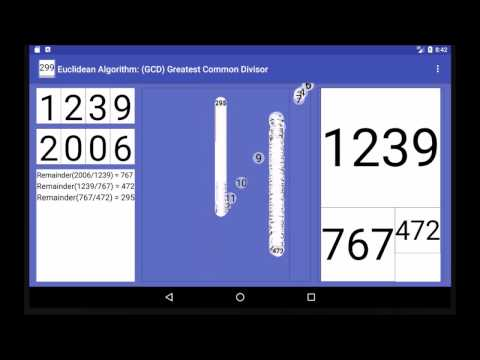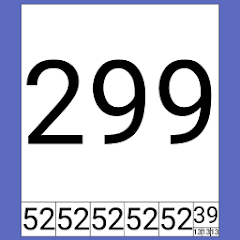# Euclidean Algorithm GCD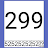1K+Everyone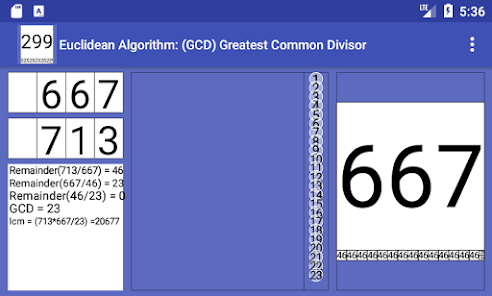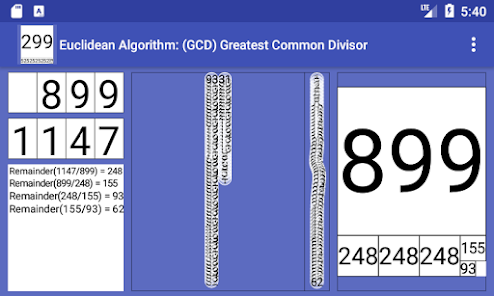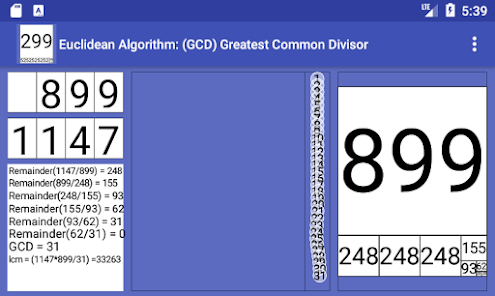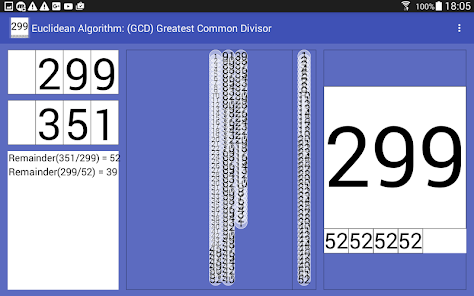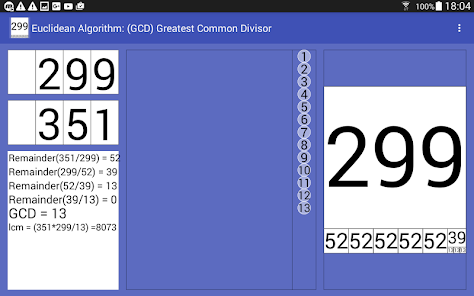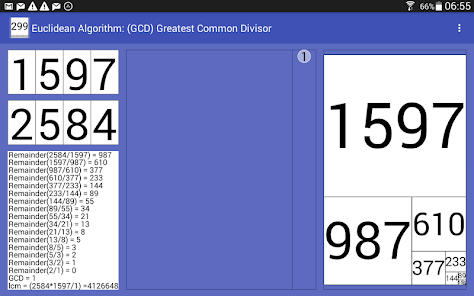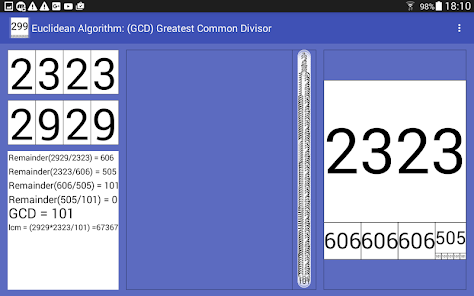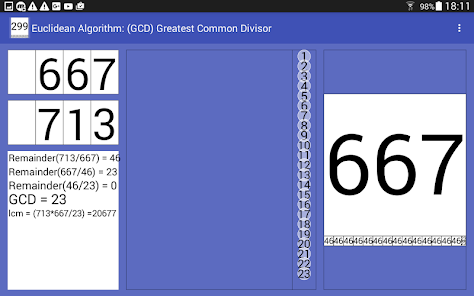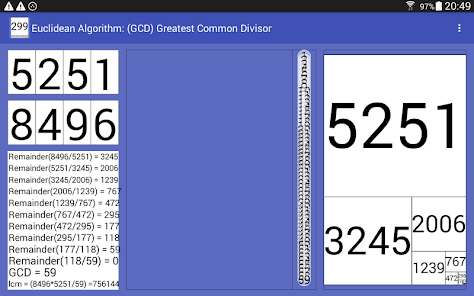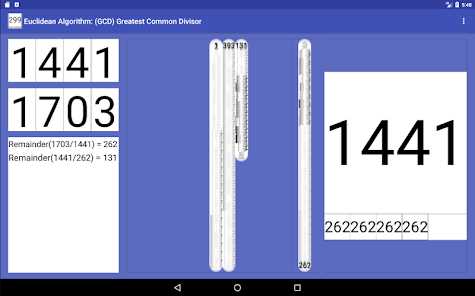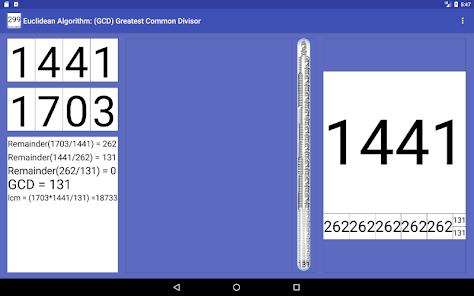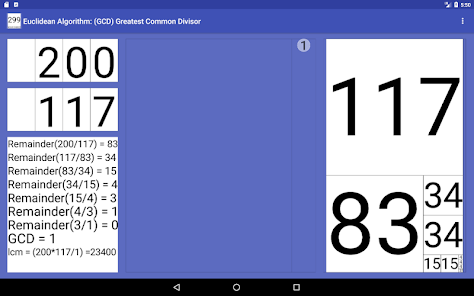Animated Euclidean Algorithm
Greatest Common Divisor.
Useful to reduce fractions

Visible Euclidean algorithm

GCD, also known as the greatest common factor (gcf), highest common factor (hcf), greatest common measure (gcm), or highest common divisor.

Dynamic and geometric representation of the algorithm.

Recursive algorithm
And Least Common Multiple deduced from GCD:
lcm(a,b) = a*b / gcd(a,b)

Useful to understand the gcd (Euclidean Algorithm) recursive code: (Java)

int gcd(int m, int n){
if(0==n){
return m;
}else{
return gcd(n,m%n);
}
}

Algorithm executed by Dandelions coming from the nearby Mathematical Garden

Euclidean Algorithm History:
("The Pulverizer")

The Euclidean algorithm is one of the oldest algorithms in common use.
It appears in Euclid's Elements (c. 300 BC), specifically in Book 7 (Propositions 1–2) and Book 10 (Propositions 2–3).
Centuries later, Euclid's algorithm was discovered independently both in India and in China, primarily to solve Diophantine equations that arose in astronomy and making accurate calendars.
In the late 5th century, the Indian mathematician and astronomer Aryabhata described the algorithm as the "pulverizer", perhaps because of its effectiveness in solving Diophantine equations.

Acknowledgements:
Joan Jareño (Creamat) (Addition of lcm)
Updated on
Oct 4, 2020

## Data safety

Safety starts with understanding how developers collect and share your data. Data privacy and security practices may vary based on your use, region, and age. The developer provided this information and may update it over time.No data shared with third parties